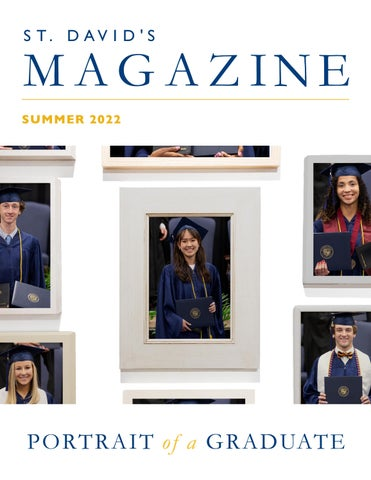# St. David's Magazine2

PURS EXCE UE PURS LLEN CE EXCE UE LLEN CE

SEEK CHRI

SEEK CHRI ST

ST PERSE V T H RO U E R E PERSE T H R O V E RCEH A L L EG H NGES CHAL UGH LENG ES

COLL A G E N E R B O R AT E COLL OUSL A G E N E B O R AT E EMBR Y RO U S L E A R AC E LY EMBR NING L E A R AC E NING

1

PUR PUR E X C SU E ELL E X C SU E ENC ELL ENC E E

SE E C H RK IS

T

SE E C H RK IS

LEA LEA H DW H U MD W IT U M IL IT IT H Y IL IT H Y

T

PER SE V PER T E T H R SE V E RC H R O U R E C H A O U G HEH A L L E G H NG LLE ES NG ES CO CO G LLAB G E NL L A B O E N E R O O R AT U SL E E R O R AT Y U SL E EMB Y R AC EMB L L E A R A C E E A R N INE R N IN G G